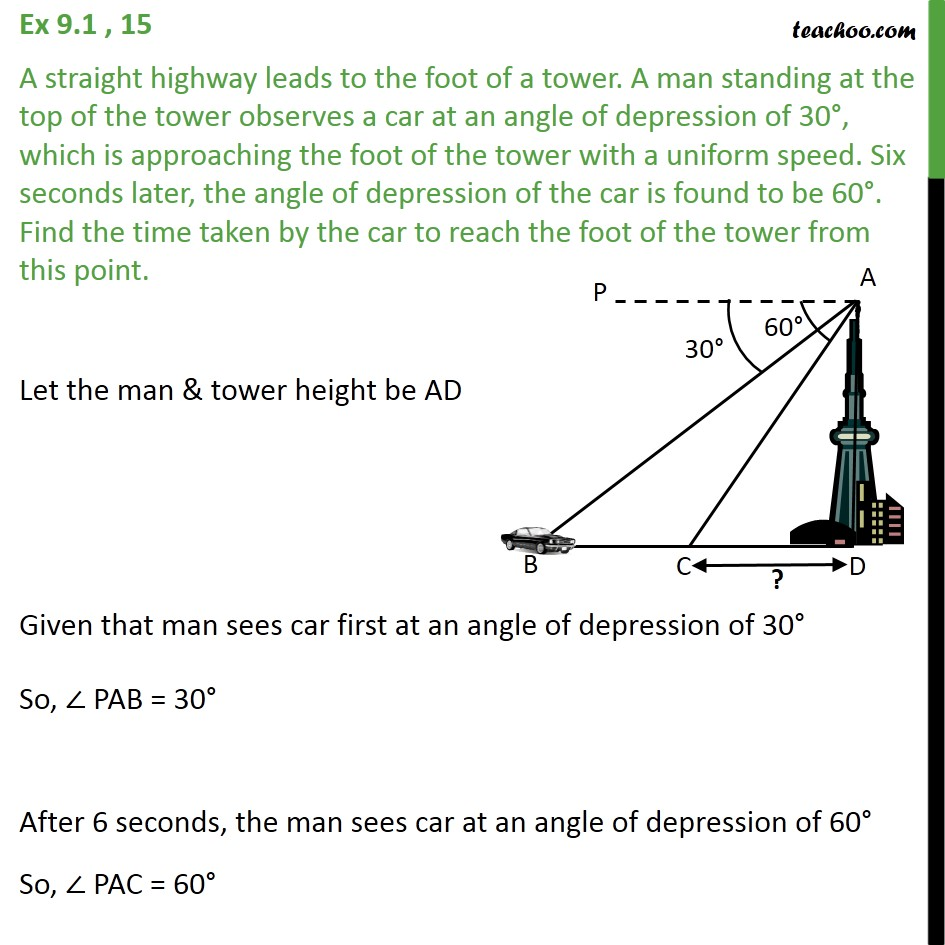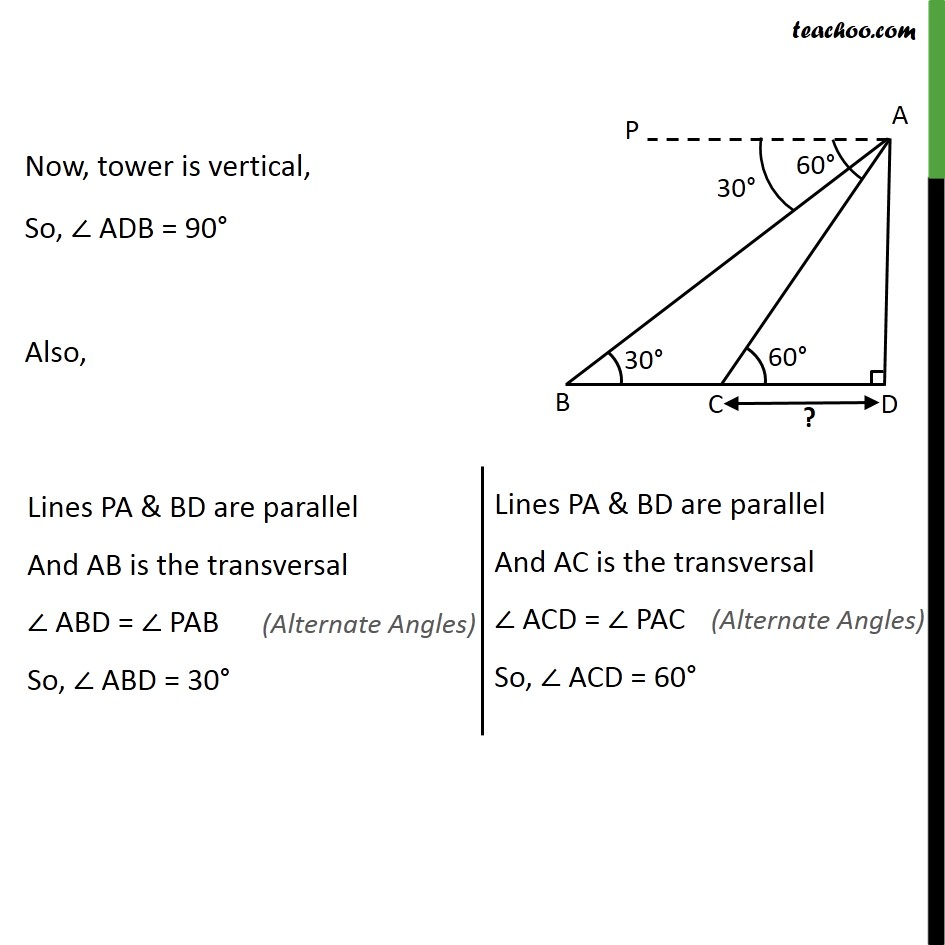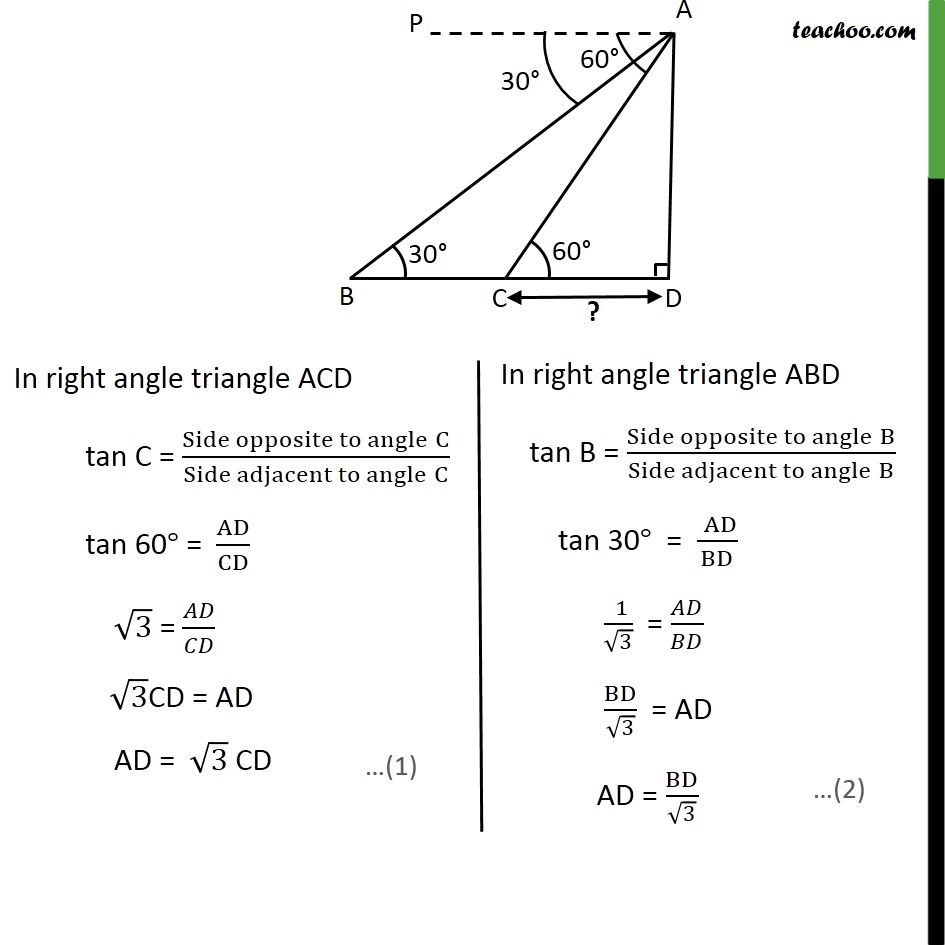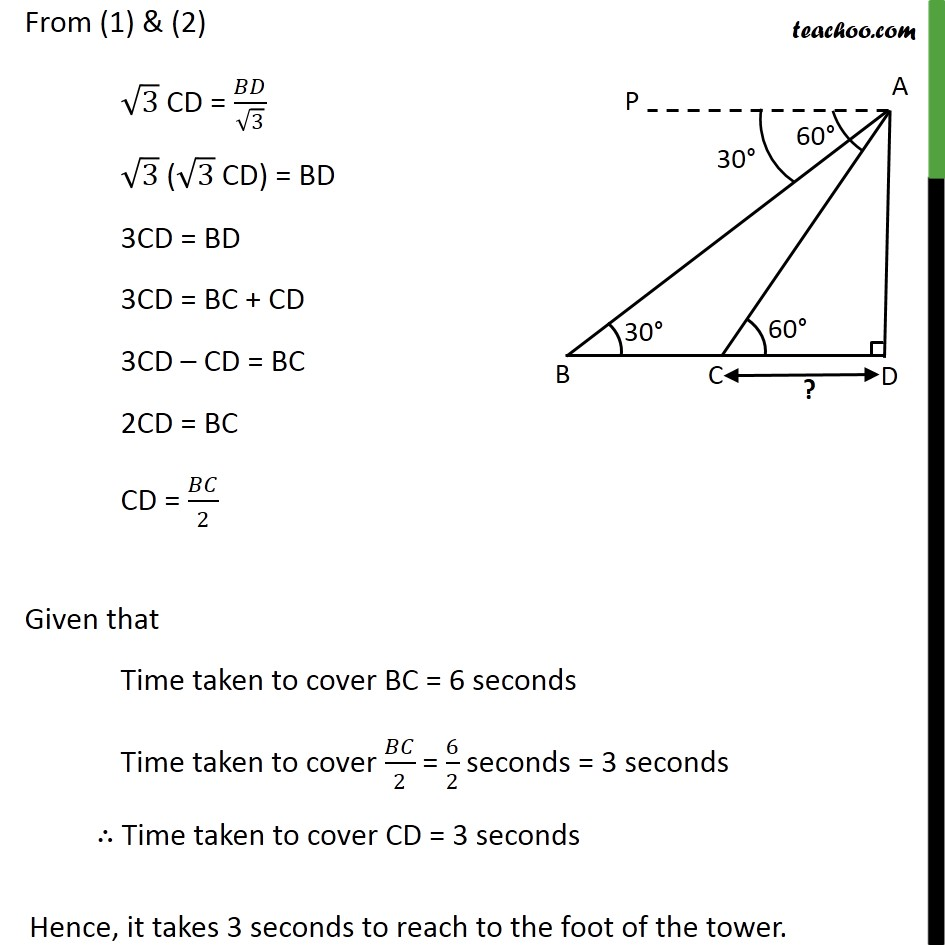Ex 9.1

Chapter 9 Class 10 Some Applications of Trigonometry
Serial order wiseLearn in your speed, with individual attention - Teachoo Maths 1-on-1 Class

### Transcript

Ex 9.1 , 15 A straight highway leads to the foot of a tower. A man standing at the top of the tower observes a car at an angle of depression of 30 , which is approaching the foot of the tower with a uniform speed. Six seconds later, the angle of depression of the car is found to be 60 . Find the time taken by the car to reach the foot of the tower from this point. Let the man & tower height be AD Given that man sees car first at an angle of depression of 30 So, PAB = 30 After 6 seconds, the man sees car at an angle of depression of 60 So, PAC = 60 Now, tower is vertical, So, ADB = 90 Also, From (1) & (2) 3 CD = / 3 3 ( 3 CD) = BD 3CD = BD 3CD = BC + CD 3CD CD = BC 2CD = BC CD = /2 Given that Time taken to cover BC = 6 seconds Time taken to cover /2 = 6/2 seconds = 3 seconds Time taken to cover CD = 3 seconds